Subsections

# Convex Formulation of the Upper Bound Approach with Noise in all Images

In this section, we propose a convex formulation of the principles sketched in section 7.2 that, compared to (144), accounts for noise in both the template and the input images. We can express this in terms of image-plane measurements. As in (164,166), our approach is formulated as an SOCP problem. However, contrary to (164,166), our approach is a point-wise method that does not require us to tune the relative influence of minimizing the reprojection error and maximizing the depths.

## Noise in the Template Only

Let us first remark that the basic principles explained in section 7.2 can be formulated as SOCP problems. In this first formulation, the noise is only accounted for in the template image. The inextensibility constraintcan be written: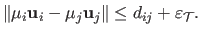(7.1)

Including the maximization of the depths, we obtain this SOCP problem:(7.2)

where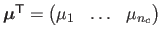, and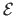is a set of pairs of points to which the inextensibility constraints are applied.

## Noise in Both the Template and the Input Images

Let us now suppose that the inaccuracies are expressed in terms of image-plane measurements. Suppose that points are measured in the image with a maximum error of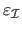, i.e.(7.3)

Since we are searching for the true 3D position of the point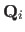, we say that: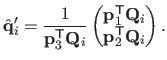(7.4)

Equation (7.3) can thus be rewritten:(7.5)

We finally add the inextensibility constraints and the maximization of the depths (which are given by) and we obtain the following SOCP problem: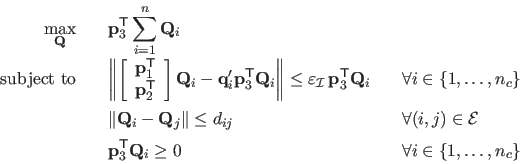(7.6)

whereis the concatenation of the 3D points, for.

Contributions to Parametric Image Registration and 3D Surface Reconstruction (Ph.D. dissertation, November 2010) - Florent Brunet
Webpage generated on July 2011
PDF version (11 Mo)